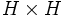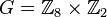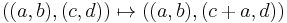# Characteristicity is not finite direct power-closed

This article gives the statement, and possibly proof, of a subgroup property (i.e., characteristic subgroup) not satisfying a subgroup metaproperty (i.e., finite direct power-closed subgroup property).
View all subgroup metaproperty dissatisfactions | View all subgroup metaproperty satisfactions|Get help on looking up metaproperty (dis)satisfactions for subgroup properties
Get more facts about characteristic subgroup|Get more facts about finite direct power-closed subgroup property|

## Statement

### Statement with symbols

It is possible to have a group$G$ and a characteristic subgroup$H$ of$G$ such that in the direct square$G \times G$, the corresponding direct square$H \times H$, viewed as a subgroup, is not a characteristic subgroup.

## Notion of finite direct power-closed characteristic

A subgroup$H$ of a group$G$ is termed a finite direct power-closed characteristic subgroup if for any natural number$n$,$H^n$ is characteristic in$G^n$.

## Proof

Further information: direct product of Z8 and Z2

Suppose$G = \mathbb{Z}_8 \times \mathbb{Z}_2$, and$H$ is the subgroup of order four given by:$H := \{ (2,1), (4,0), (6,1), (0,0) \}$

Note that this subgroup is characteristic but not fully invariant, and is a standard example of the fact that characteristic not implies fully invariant in finite abelian group.

Then, we claim that$H \times H$ is not a characteristic subgroup inside$G \times G$. To see this, consider the endomorphism of$G$ that projects onto the$\mathbb{Z}_8$ coordinate, i.e., the map:$\pi: (a,b) \mapsto (a, 0)$

Now, consider the automorphism of$G \times G$ defined as:$(x,y) \mapsto (x, y + \pi(x))$

This is an endomorphism as it is a sum of endomorphisms; it is an automorphism because it has an inverse:$(x,y) \mapsto (x, y - \pi(x))$

In full, the automorphism is:$((a,b),(c,d)) \mapsto ((a,b),(c + a,d))$

Under this automorphism, the element:$((2,1),(2,1)) \in H \times H$

gets mapped to the element:$((2,1),(4,1))$

which is not inside$H \times H$.